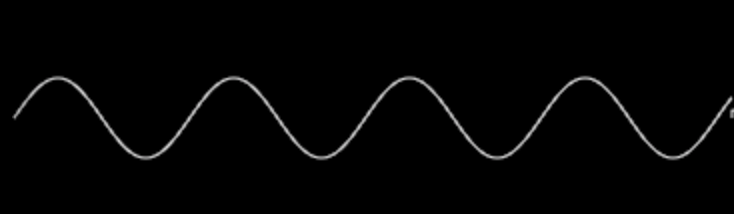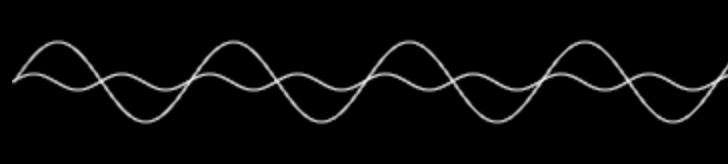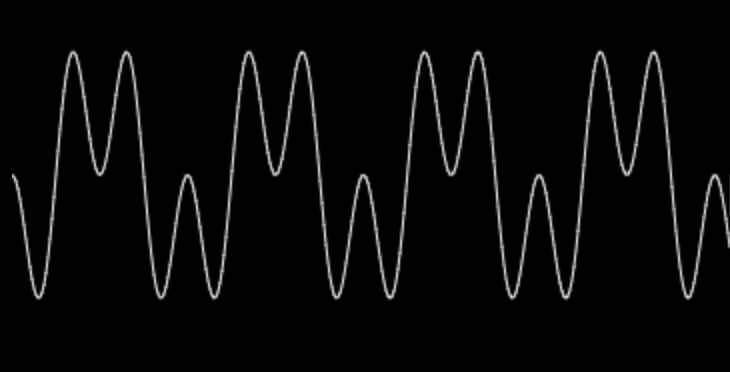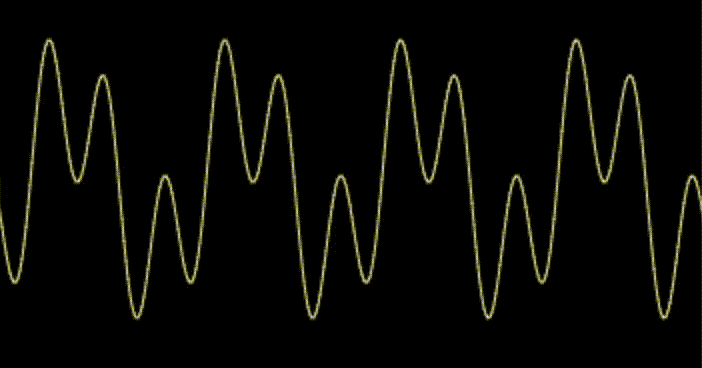# Sine Wave Animation

## Background

I have been learning a creative coding field as my personal project. Whenever I saw wonderful graphics with motion, they always intrigues me and drive me to write such creative codes. However, creating cool animation is not easy for me. I realised what I have to learn at first. That’s a basic knowledge of sin and cos algorithms. In this post I explain how the animation by sin wave is changing.

## Draw Basic Sin Wave

First of all, I researched on how sin wave draws.

https://codepen.io/mitsuya_bauhaus/pen/PoqrYwQ

As you can see above code, sine wave consists of a large number of dots. The main code is:

for (let i = 0; i < window.innerWidth / 2; i += step) {
ctx.beginPath();
const x = i;
const y = wave.y + Math.sin(i * wave.length) * wave.amplitude;
ctx.ellipse(x, y, 2, 2, Math.PI / 4, 0, 2 * Math.PI);
ctx.stroke();
}

The more step variable is smaller, the closer the distance between dots are, which becomes single line as a sine wave. In short, to make sine wave, what we need is to drop dots along with mathematic sine method. Then instead of dropping dots, if we connect between the dropping dots so that making line, sine wave is created. This is a basic logic to draw sine wave.

## Simple Animation

How can we create a sine curve animation? It is so simple. Just update theta value passing into sine method that is to generate sine curve on every frame.

In this case, I realised two types for animating sine wave. The one is normally sliding with keeping the shape of sine wave. The second is a bit curious. While animating, the amplitude of sine wave is repeating up and down.

https://codepen.io/mitsuya_bauhaus/pen/MWwdRxR

The worth point to note is that the sine wave function to generate sine wave is multiplied by another sine wave function.

wave.y + Math.sin(i * wave.length + theta) * wave.amplitude * Math.sin(theta)

## Complicate Wave Patterns

https://codepen.io/mitsuya_bauhaus/pen/WNvBBVN

To combine over 2 sin waves gets deformed single sine wave. The logic is clear once You understood the mechanism. For example, there are two sine waves with same theta value below.If the two waves overlaid in a same canvas, the graphic would illustrate like below image.These waves are eventually mixed as a single wave. The logic is that two points on the same y-axis are composited then new point is yielded by the added points. Each point follows the same calculation flow. Then complicated sine wave is created at the end.This static sine wave can also animate by updating theta value in every frame.## Conclusion

These sine wave consist of just two waves but if you apply many sine waves, you get a more interesting wave like a noise wave.
Hoping this post is helpful for some developers and I implemented sine animations as effects in my portfolio. Please check it out.

https://mitsuyacider.com/

#### You may also like